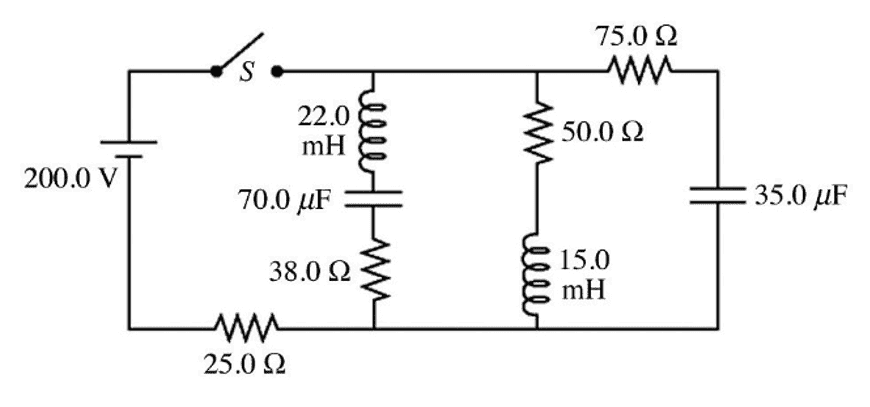# Potential Drop Across 15-mH Inductor at t=0?

• Truman I
In summary, current flows through the circuit as long as the switch is closed. If the switch is open for a long time, the potential drop across the 15-mH inductor will occur.f

#### Truman I

Homework Statement
1) For the circuit shown in the figure, the switch has been open for a very long time.
(a) What is the potential drop across the 15.0-mH inductor just after closing the switch?
Relevant Equations
ε = L*(di/dt)
V_R = iRAt t=0, I believe that the current is instantaneously 0 Amps. If that is correct, then technically at that instant there is no voltage drop across any of the resistors due to Ohm's Law. So I replaced the resistors with wire. Next, I tried replacing all of the capacitors with open circuits to simplify everything further. When I did that, there was only one closed loop for the current to travel through, and that's the loop with my 15-mH inductor. But that's where I'm getting lost.

My only other thought is that I need to use the equation ε = L*(di/dt) but there are two unknowns. I want to find ε for the 15 mH inductor, but I don't know di/dt.

At t=0, I believe that the current is instantaneously 0 Amps.
Based on what, precisely ?
You have a voltage source with an internal resistance of 25 ##\Omega## and three branches. From left to right LCR, RL and RC.
If that is correct, then technically at that instant there is no voltage drop across any of the resistors due to Ohm's Law
It is certainly not correct for the rightmost branch. Check RC circuit under: Time considerations. The capacitor will instantaneously start charging with a current of 1 A. And the 75 ##\Omega## and 25 ##\Omega## will have a voltage drop of 150 V and 50 V, respectively
I tried replacing all of the capacitors with open circuits to simplify everything further
That's not an argument ! The capacitors are uncharged and if they are connected to a voltage source, they start charging to the tune of ##\displaystyle{I = C{dV\over dt}}\ ##. I.e. instantaneously they are short-circuits, not open circuits !

Now check RL circuits under : Time considerations and try again.

##\ ##

Homework Statement:: 1) For the circuit shown in the figure, the switch has been open for a very long time.
(a) What is the potential drop across the 15.0-mH inductor just after closing the switch?
Relevant Equations:: ε = L*(di/dt)
V_R = iR

At t=0, I believe that the current is instantaneously 0 Amps.
The current where? Remember that the 35 μF capacitor is initially uncharged and as soon as the switch is closed it acts as a "short". Redraw the circuit taking that into account.

It is certainly not correct for the rightmost branch. Check RC circuit under: Time considerations. The capacitor will instantaneously start charging with a current of 1 A. And the 75 ##\Omega## and 25 ##\Omega## will have a voltage drop of 150 V and 50 V, respectively

Yeah I definitely had a misconception about current there. So at t=0, the 75 ohm and 25 ohm resistors act like they are in series. 150 Volts will drop across the 75 ohm resistor (Ohm's Law). Therefore, because the 15-mH Inductor is in parallel with the 75 ohm resistor, there will also be a potential difference of 150 volts.

Is that logic correct?

•BvU
Yeah I definitely had a misconception about current there. So at t=0, the 75 ohm and 25 ohm resistors act like they are in series. 150 Volts will drop across the 75 ohm resistor (Ohm's Law). Therefore, because the 15-mH Inductor is in parallel with the 75 ohm resistor, there will also be a potential difference of 150 volts.

Is that logic correct?
•